# Asymptotes

Definition. The line $x=a$ is called vertical asymptote of the curve $y=f{{\left({x}\right)}}$ if at least one of the following statements is true:

1. $\lim_{{{x}\to{a}}}{f{{\left({x}\right)}}}=\infty$
2. $\lim_{{{x}\to{{a}}^{+}}}{f{{\left({x}\right)}}}=\infty$
3. $\lim_{{{x}\to{{a}}^{{-}}}}{f{{\left({x}\right)}}}=\infty$
4. $\lim_{{{x}\to{a}}}{f{{\left({x}\right)}}}=-\infty$
5. $\lim_{{{x}\to{{a}}^{+}}}{f{{\left({x}\right)}}}=-\infty$
6. $\lim_{{{x}\to{{a}}^{{-}}}}{f{{\left({x}\right)}}}=-\infty$

For example y-axis $\left({x}={0}\right)$ is a vertical asymptote of the curve ${y}=\frac{{1}}{{{x}}^{{2}}}$ because $\lim_{{{x}\to{0}}}\frac{{1}}{{{x}}^{{2}}}=\infty$.

Geometrically vertical asymptote is vertical line to which approaches graph of the function but never intersects it. As function approaches ${a}$ values of function become so large, that we can't draw them.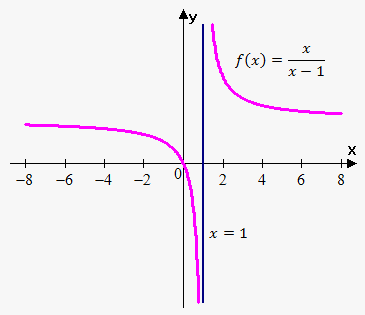Example 1. Find vertical asymptotes of ${f{{\left({x}\right)}}}=\frac{{x}}{{{x}-{1}}}$.

In Limits Involving Infinity note we saw that if $\lim_{{{x}\to{{a}}^{+}}}{f{{\left({x}\right)}}}={L}\ne{0}$ and $\lim_{{{x}\to{a}+}}{g{{\left({x}\right)}}}={0}$ then $\lim_{{{x}\to{{a}}^{+}}}{\left(\frac{{f{{\left({x}\right)}}}}{{g{{\left({x}\right)}}}}\right)}=\infty$ (Law 8 for one-sided limits).

So, to find points where function approach infinity, we need to find points where denominator equals 0, and make sure that numerator doesn't equal 0 at this point.

The only point where denominator equals 0 is point 1, and numerator doesn't equal 0 at this point.

Let's find out one-sided limits at this point. When ${x}$ approaches 1 from the right then ${x}-{3}$ becomes very small positive value and ${x}$ approaches 1. Thus, $\lim_{{{x}\to{{1}}^{+}}}\frac{{{x}}}{{{x}-{1}}}=\infty$ When ${x}$ approaches 1 from the left then ${x}-{1}$ becomes very small negative value and ${x}$ approaches 1. Thus, $\lim_{{{x}\to{{1}}^{{-}}}}\frac{{{x}}}{{{x}-{1}}}=-\infty$. So, we showed that both one-sided limits are infinite. In fact it was sufficiently to show that at least one of the limits is infinite.

Thus, ${x}={1}$ is vertical asympote.

In fact function can have more than vertical asymptote.

Example 2. Find vertical asymptotes of ${y}={\tan{{\left({x}\right)}}}$.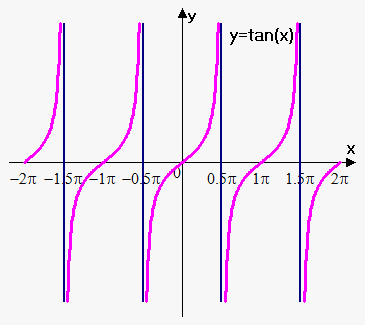Recall that by definition ${\tan{{\left({x}\right)}}}=\frac{{{\sin{{\left({x}\right)}}}}}{{{\cos{{\left({x}\right)}}}}}$.

This means that there will be vertical asymptotes at points where ${\cos{{\left({x}\right)}}}={0}$, i.e. points of the form ${x}=\frac{\pi}{{2}}+\pi{k},{k}\in\mathbb{Z}$.

Therefore, ${\tan{{\left({x}\right)}}}$ has infinitely many asymptotes.

Example 3. Find vertical asymptotes of ${f{{\left({x}\right)}}}=\frac{{{{x}}^{{2}}-{x}}}{{{{x}}^{{2}}+{x}-{2}}}$.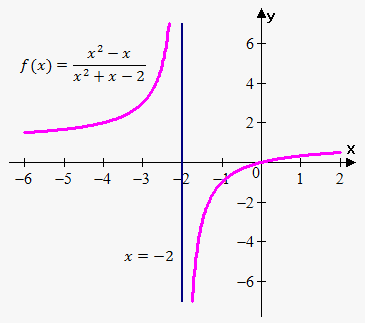Since ${{x}}^{{2}}+{x}-{2}={\left({x}+{2}\right)}{\left({x}-{1}\right)}$ then denominator equals 0 when ${x}=-{2}$ and ${x}={1}$.

However, at point ${x}={1}$ numerator also equals 0. We need to additionally check this point.So, $\lim_{{{x}\to{1}}}\frac{{{{x}}^{{2}}-{x}}}{{{{x}}^{{2}}+{x}-{2}}}=\lim_{{{x}\to{1}}}\frac{{{x}{\left({x}-{1}\right)}}}{{{\left({x}+{2}\right)}{\left({x}-{1}\right)}}}=$

$=\lim_{{{x}\to{1}}}\frac{{x}}{{{x}+{2}}}=\frac{{1}}{{{1}+{2}}}=\frac{{1}}{{3}}$.

Thus, there is only one vertical asymptote ${x}=-{2}$. This example showed, that you should be very cautious: you should check whether numerator equals 0 at points where denominator equals 0.

Definition. Line ${y}={L}$ is called horizontal asymptote of the function ${f{{\left({x}\right)}}}$ if either $\lim_{{{x}\to\infty}}{f{{\left({x}\right)}}}={L}$ or $\lim_{{{x}\to-\infty}}{f{{\left({x}\right)}}}={L}$.

Example 4. Find horizontal asymptotes of ${y}={1}+\frac{{1}}{{x}}$.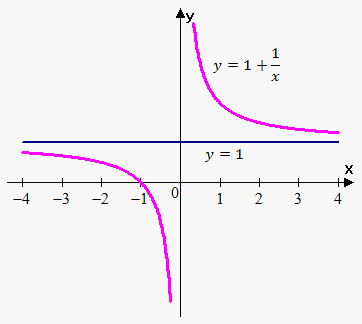Since $\lim_{{{x}\to\infty}}{\left({1}+\frac{{1}}{{x}}\right)}={1}$ then line ${y}={1}$ is horizontal asymptote.

In fact also $\lim_{{{x}\to-\infty}}{\left({1}+\frac{{1}}{{x}}\right)}={1}$, but it suffices one limit to state that ${y}={1}$ is horizontal asymptote.

In general function can have more than one horizontal asymptote.

Example 5. Find horizontal asymptotes of ${y}={\operatorname{arctan}{{\left({x}\right)}}}$.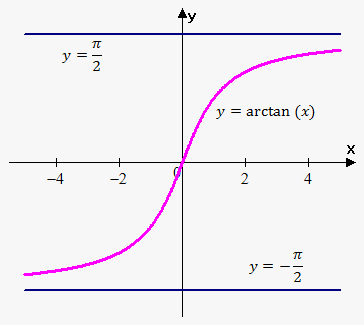Since $\lim_{{{x}\to\infty}}{\operatorname{arctan}{{\left({x}\right)}}}=\frac{\pi}{{2}}$ and $\lim_{{{x}\to-\infty}}{\operatorname{arctan}{{\left({x}\right)}}}=-\frac{\pi}{{2}}$ then there are two vertical asymptotes: ${y}=\frac{\pi}{{2}}$ and ${y}=-\frac{\pi}{{2}}$.

Definition. Line ${y}={m}{x}+{b}$ is called slant (oblique) asymptote of function ${y}={f{{\left({x}\right)}}}$ if either $\lim_{{{x}\to\infty}}{\left({f{{\left({x}\right)}}}-{\left({m}{x}+{b}\right)}\right)}={0}$ or $\lim_{{{x}\to-\infty}}{\left({f{{\left({x}\right)}}}-{\left({m}{x}+{b}\right)}\right)}={0}$.

We calculate ${m}$ as follows: ${m}=\lim_{{{x}\to\infty}}\frac{{{f{{\left({x}\right)}}}}}{{x}}{\left({m}=\lim_{{{x}\to-\infty}}\frac{{{f{{\left({x}\right)}}}}}{{x}}\right)}$.

If this limit is not finite or doesn't exist then there is no oblique asymptote.

${b}$ is calculated as follows: ${b}=\lim_{{{x}\to\infty}}{\left({f{{\left({x}\right)}}}-{m}{x}\right)}{\left({b}=\lim_{{{x}\to-\infty}}{\left({f{{\left({x}\right)}}}-{m}{x}\right)}\right)}$.

Example 6. Find all asymptotes of ${y}={x}+\frac{{1}}{{x}}$.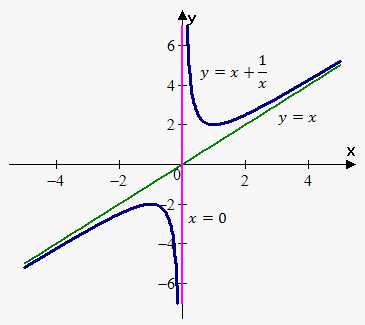There are no horizontal asymptotes because $\lim_{{{x}\to\infty}}{\left({x}+\frac{{1}}{{x}}\right)}=\infty$ and $\lim_{{{x}\to-\infty}}{\left({x}+\frac{{1}}{{x}}\right)}=-\infty$.

There is vertical asymptote ${x}={0}$ because $\lim_{{{x}\to{{0}}^{+}}}{\left({x}+\frac{{1}}{{x}}\right)}=\infty$.

This function also has oblique asymptote. Indeed, ${m}=\lim_{{{x}\to\infty}}\frac{{{x}+\frac{{1}}{{x}}}}{{x}}=\lim_{{{x}\to\infty}}{\left({1}+\frac{{1}}{{{x}}^{{2}}}\right)}={1}$.

So, ${m}={1}$. Now, ${b}=\lim_{{{x}\to\infty}}{\left({x}+\frac{{1}}{{x}}-{1}\cdot{x}\right)}=\lim_{{{x}\to\infty}}\frac{{1}}{{x}}={0}$. So, ${b}={0}$.

Therefore, slant asymptote is ${y}={x}$. On the figure to the right blue line is vertical asymptote ${x}={0}$, green line is slant asymptote ${y}={x}$.

Note, that we will obtain same slant asymptote when ${x}\to-\infty$.

In general, it is a good practice to treat cases ${x}\to\infty$ and ${x}\to-\infty$ separately.